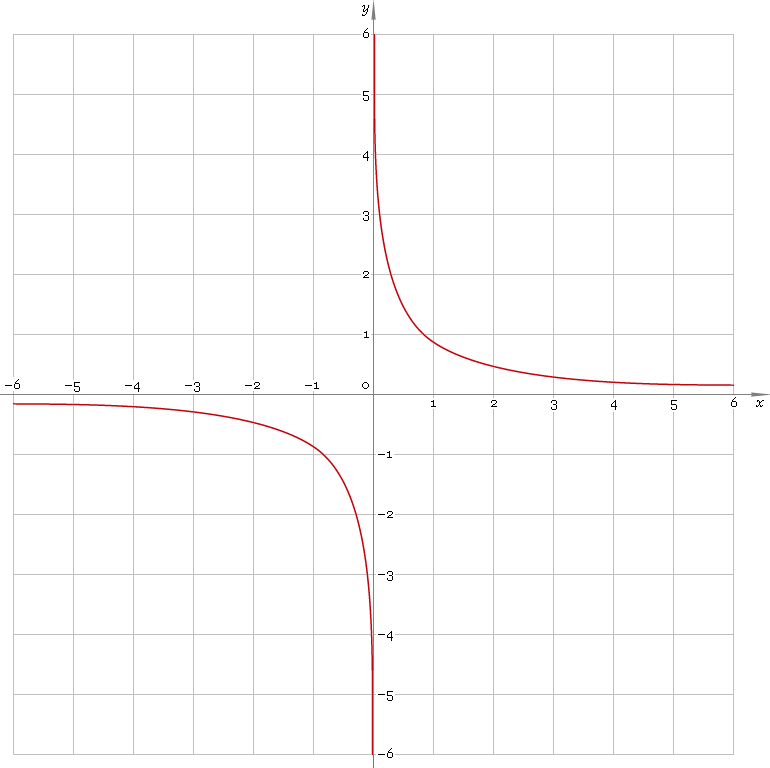The Art of Interface

# arcsch — arc-hyperbolic cosecant function

Category. Mathematics.

Abstract. Arc-hyperbolic cosecant: definition, plot, properties and identities.

## 1. Definition

Arc-hyperbolic cosecant is inverse of hyperbolic cosecant function. With the help of natural logarithm it can be represented as:

arcschx ≡ ln[1/x + √(1/x2 + 1)]

## 2. Plot

Arc-hyperbolic cosecant is antisymmetric function defined everywhere on real axis, except its singular point 0 — so, its domain is (−∞, 0)∪(0, +∞). Function plot is depicted below — fig. 1.Fig. 1. Plot of the arc-hyperbolic cosecant function y = arcschx.

Function codomain is entire real axis, except 0: (−∞, 0)∪(0, +∞).

## 3. Identities

Property of antisymmetry:

arcsch−x = −arcschx

Reciprocal argument:

arcsch(1/x) = arsinhx

Sum and difference:

arcschx + arcschy = arcsch{xy / [x√(1 + 1 /x2) + y√(1 + 1 /y2)]}
arcschx − arcschy = arcsch{xy / [y√(1 + 1 /y2) − x√(1 + 1 /x2)]}

## 4. Support

Arc-hyperbolic cosecant function arcsch of the real argument is supported by free version of the Librow calculator.

Arc-hyperbolic cosecant function arcsch of the complex argument is supported by professional version of the Librow calculator.

## 5. How to use

To calculate arc-hyperbolic cosecant of the number:

``arcsch(-1);``

To calculate arc-hyperbolic cosecant of the current result:

``arcsch(rslt);``

To calculate arc-hyperbolic cosecant of the number x in memory:

``arcsch(mem[x]);``